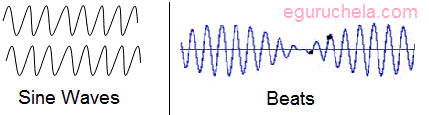# Beats

When two sound waves of different frequency approach your ear, the alternating constructive and destructive interference causes the sound to be alternatively soft and loud - a phenomenon which is called "beating" or producing beats.a beat is an interference pattern between two sounds of slightly different frequencies, perceived as a periodic variation in volume whose rate is the difference of the two frequencies.

### Beat Frequency

There are two wave of frequency ω1 and ω2.Then the beat frequency will be ω1 - ω2.

The wave of frequency is denoted by ω

Also ω = 2πν

Therefore νbeat = ν1 – ν2Mathematically beats can be understood from a mathematical identity for sine waves. For sounds with (angular) frequencies of w1 and w2 added together the identity gives

sin(w1t) + sin(w2t) = 2 sin(w3t)cos( w4t)

where w3 is the average of w1 and w2

and w4 is one half of their difference.

If the difference frequency is small, the sound is the sound of a single tone at the average frequency, but with a volume which varies in time at the difference frequency. This is illustrated on the graphic to the right. The sum is a sine wave with a variable amplitude and the pattern repeats every 0.05s. That is, there are 1/0.05 = 20 repeats per second.